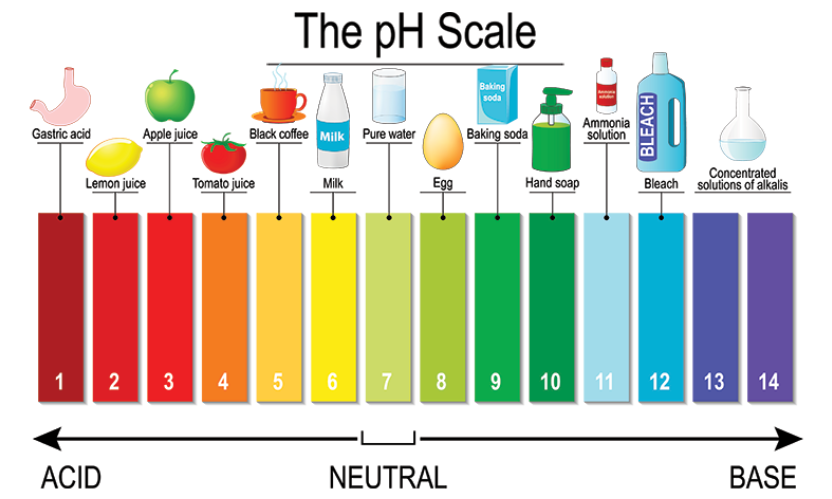# How the pH of distilled water changes from 7 to 6.14 while boiling it at 100oC ?

• pH decreases with increase in temperature. This does not mean that the water becomes more acidic at higher temperatures.
• A solution is considered as acidic if there is excess of hydrogen ions ( H+) over hydroxide ions (OH-).
• In case of pure water, the concentration of hydrogen ions and hydroxide ions are same and hence, the water is still neutral (even if its pH changes).
• Therefore, at 100°C, a pH value of 6.14 is actually the new neutral point on the pH scale at this higher temperature.

(Refer image for better understanding)(The pH of a solution is a measure of the molar concentration of hydrogen ions in the solution and as such is a measure of the acidity or basicity of the solution.  The letters pH stand for "power of hydrogen" and the numerical value for pH is just the negative of the power of 10 of the molar concentration of H+ ions.)

Updated on: 10-Oct-2022

59 Views